# Bound variable

(diff) ← Older revision | Latest revision (diff) | Newer revision → (diff)

bound occurrence of a variable

A type of occurrence of a variable in a linguistic expression. An exact definition for each formalized language depends on the rules of formation of the language. It is not possible to substitute objects in place of a bound variable, since such a substitution would lead to meaningless expressions. But the replacement of a bound variable, where it occurs, by a new (for a given expression) variable leads to an expression with the same meaning. For example, in the expressionsis a bound variable. Replacingby a number leads to a meaningless expression, whereas by writingeverywhere instead ofone obtains an expression with exactly the same meaning.

Bound variables always arise in applying to an expressionwith free occurrences of a variablean operator variable(see Free variable). In the resulting expression, all the occurrences ofinthat were previously free become bound. We mention below certain operators that are often used (next to the operators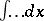and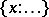), in whichis an operator variable: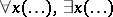, that is, the universal and existential quantifiers;, that is, a definite integral with respect to;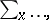that is, summation over;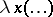, that is, a function ofthe value of which atis. Specific linguistic expressions can be substituted in place of the dots.

In real (non-formalized) mathematical texts it is possible to have a non-unique use for one and the same expression; in this connection distinguishing a bound variable in a given expression depends on the context and meaning of the expression. In formalized languages there is a formal procedure for distinguishing free and bound occurrences of variables.

How to Cite This Entry:
Bound variable. Encyclopedia of Mathematics. URL: http://encyclopediaofmath.org/index.php?title=Bound_variable&oldid=14900
This article was adapted from an original article by V.N. Grishin (originator), which appeared in Encyclopedia of Mathematics - ISBN 1402006098. See original article